# The Basic Concept Of Magnets And Magnetic Force

This quiz contains questions related to the concept of magnets. Different types of questions related to the magnetic forces are asked in the quiz to clear the concept of magnetic forces for a student. The students are asked to find the direction of force when two magnets are placed near each other with different positions.

Start Quiz

Two magnets are shown below.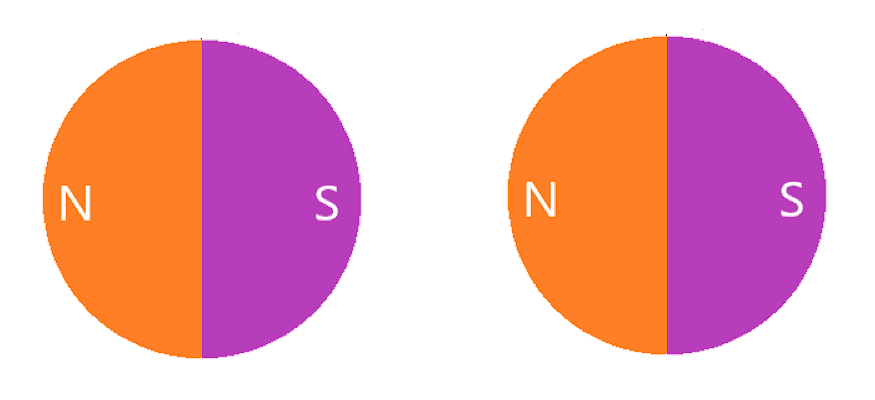Will these magnets attract or repel each other?

Attract

Repel

Two magnets are shown below.Will these magnets attract or repel each other?

Attract

Repel

Two magnets are shown below.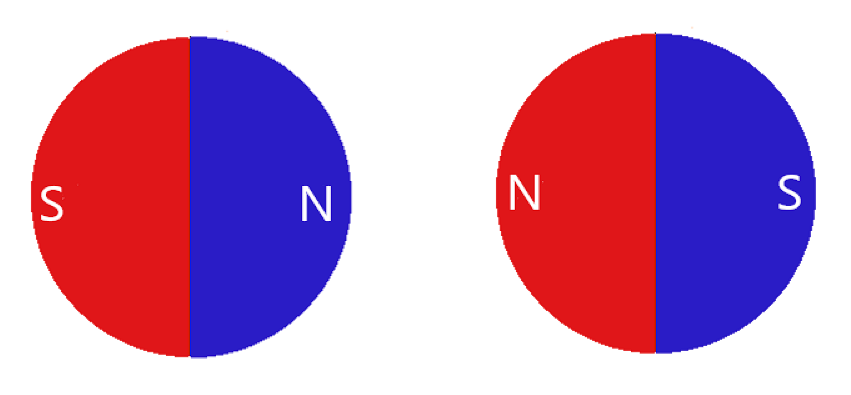Will these magnets attract or repel each other?

Attract

Repel

Two magnets are shown below.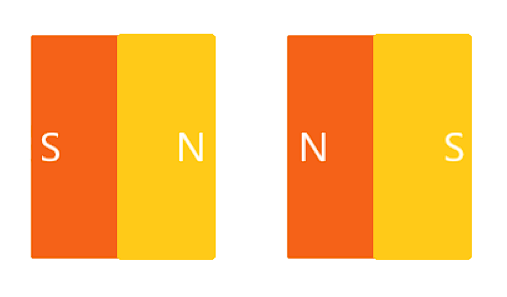Will these magnets attract or repel each other?

Attract

Repel

Two magnets are shown below.Will these magnets attract or repel each other?

Attract

Repel

Two magnets are shown below.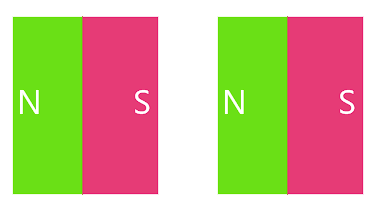Will these magnets attract or repel each other?

Attract

Repel

The two magnets shown below attract each other.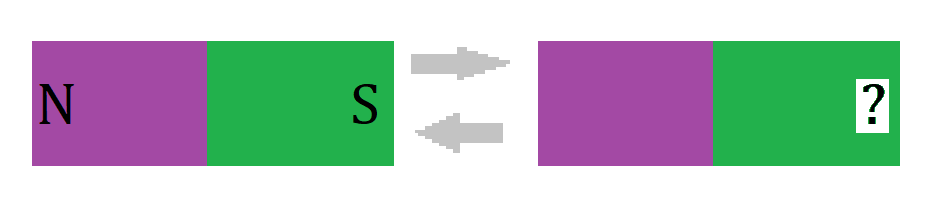Find the missing pole shown in the figure.

North Pole

South Pole

The two magnets shown below repel each other.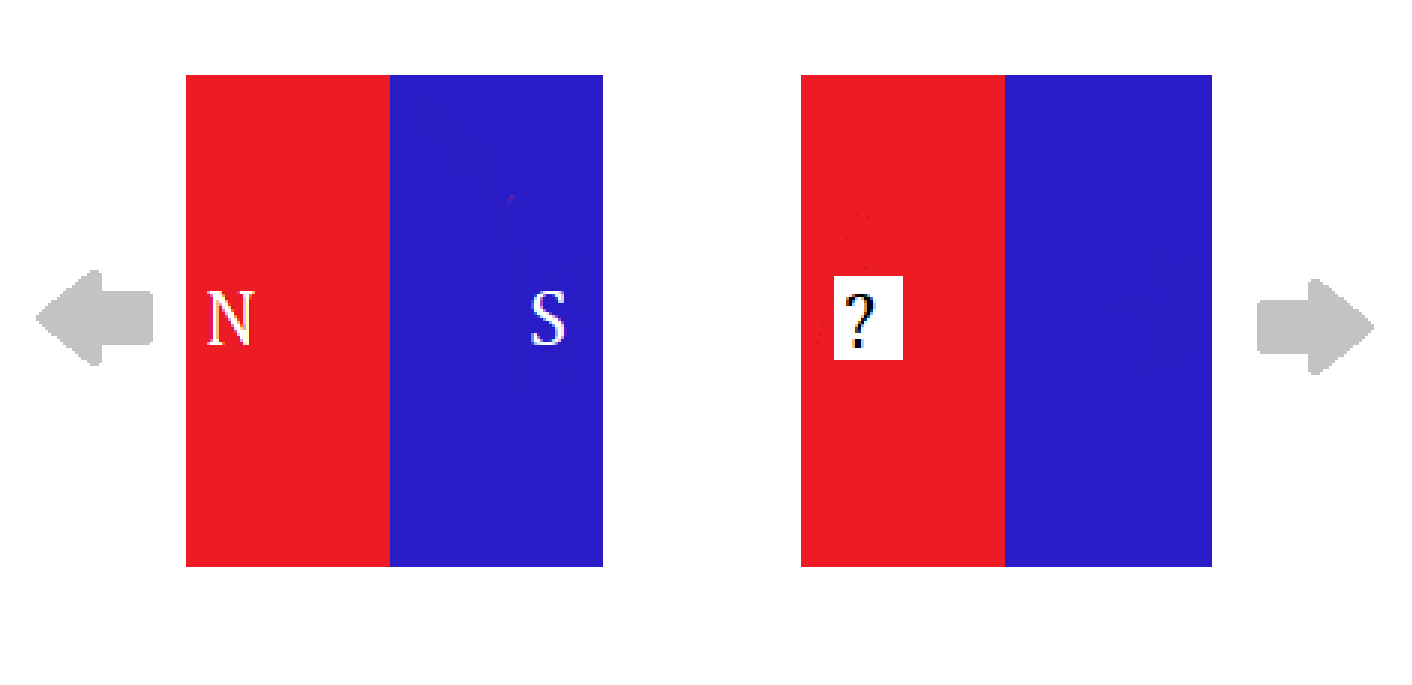Find the missing pole shown in the figure.

North Pole

South Pole

Quiz/Test Summary
Title: The Basic Concept Of Magnets And Magnetic Force
Questions: 8
Contributed by: